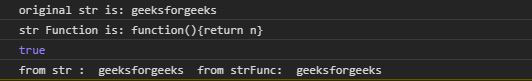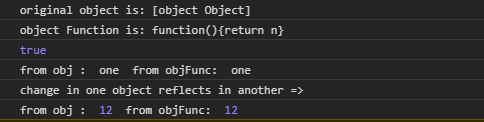Open in App
Not now

# Underscore.js _.constant() Function

• Last Updated : 10 Jul, 2020

Underscore.js is a JavaScript library that makes operations on arrays, string, objects much easier and handy. The _.constant() function is used to create a function that returns the parameter given to it. It is almost same as _.identity() function.

Note: It is very necessary to link the underscore CDN before going and using underscore functions in the browser. When linking the underscore.js CDN, the “_” is attached to the browser as global variable.

Syntax:

`_.constant( object );`

Parameters: This function accepts single parameter object.

Return Value: This function returns the parameter as given to the function.

Below examples illustrate the _.constant() function in Underscore.js:

Example 1:

 ```<``html``>`` ` `<``head``>``    ``<``script` `src``=``"https://cdnjs.cloudflare.com/ajax/libs/underscore.js/1.9.1/underscore-min.js"``>``    `````` ` `<``body``>``    ``<``script``>`` ` `        ``let str = "geeksforgeeks"`` ` `        ``// _.constant function of underscore.js``        ``let strFunc = _.constant(str);``        ``console.log(`original str is: \${str}`)``        ``console.log(`str Function is: \${strFunc}`)`` ` `        ``// This will return true``        ``console.log(str === strFunc())`` ` `        ``// Both strings are exactly same``        ``console.log("from str : ", str, ``            ``" from strFunc: ", strFunc());``    `````` ` ``

Output:Example 2:

 ```<``html``>`` ` `<``head``>``    ``<``script` `src``=``"https://cdnjs.cloudflare.com/ajax/libs/underscore.js/1.9.1/underscore-min.js"``>``    `````` ` `<``body``>``    ``<``script``>`` ` `        ``// Creating a object``        ``let obj = {``            ``"a": "one",``            ``"b": "two",``            ``"c": "three"``        ``}`` ` `        ``// _.constant function of underscore.js``        ``let objFunc = _.constant(obj);``        ``console.log(`original object is: \${obj}`)``        ``console.log(`object Function is: \${objFunc}`)`` ` `        ``// This will return true``        ``console.log(obj === objFunc())`` ` `        ``// Both objects are exactly same``        ``console.log("from obj : ", obj.a, ``            ``" from objFunc: ", objFunc().a);`` ` `        ``// Made Changes in object``        ``obj.a = 12`` ` `        ``// Change in one object reflects``        ``// in another``        ``console.log("change in one object "``                ``+ "reflects in another =>")``                 ` `        ``console.log("from obj : ", obj.a, ``            ``" from objFunc: ", objFunc().a);``    `````` ` ``

Output:My Personal Notes arrow_drop_up